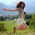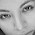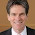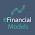Monday, March 21, 2016

The Habit Habit

The Habit Habit. This is an essay expanding slightly on a talk I gave at the University of Melbourne's excellent "Finance Down Under" conference. The slides

(Note: This post uses mathjax for equations and has embedded graphs. Some places that pick up the post don't show these elements. If you can't see them or links come back to the original. Two shift-refreshes seem to cure Safari showing "math processing error".)

Habit past: I start with a quick review of the habit model. I highlight some successes as well as areas where the model needs improvement, that I think would be productive to address.

Habit present: I survey of many current parallel approaches including long run risks, idiosyncratic risks, heterogenous preferences, rare disasters, probability mistakes -- both behavioral and from ambiguity aversion -- and debt or institutional finance. I stress how all these approaches produce quite similar results and mechanisms. They all introduce a business-cycle state variable into the discount factor, so they all give rise to more risk aversion in bad times. The habit model, though less popular than some alternatives, is at least still a contender, and more parsimonious in many ways,

Habits future: I speculate with some simple models that time-varying risk premiums as captured by the habit model can produce a theory of risk-averse recessions, produced by varying risk aversion and precautionary saving, as an alternative to  Keynesian flow constraints or new Keynesian intertemporal substitution. People stopped consuming and investing in 2008 because they were scared to death, not because they wanted less consumption today in return for more consumption tomorrow.

Throughout, the essay focuses on challenges for future research, in many cases that seem like low hanging fruit. PhD students seeking advice on thesis topics: I'll tell you to read this. It also may be useful to colleagues as a teaching note on macro-asset pricing models. (Note, the parallel sections of my coursera class "Asset Pricing" cover some of the same material.)

I'll tempt you with one little exercise taken from late in the essay.

A representative consumer with a fixed habit $$x$$ lives in a permanent income economy, with endowment $$e_0$$ at time 0 and random endowment $$e_1$$ at time 1. With a discount factor $$\beta=R^f=1$$, the problem is

$\max\frac{(c_{0}-x)^{1-\gamma}}{1-\gamma}+E\left[ \frac {(c_{1}-x)^{1-\gamma}}{1-\gamma}\right]$ $c_{1} = e_{0}-c_{0} +e_{1}$ $e_{1} =\left\{ e_{h},e_{l}\right\} \; pr(e_{l})=\pi.$ The solution results from the first order condition $\left( c_{0}-x\right) ^{-\gamma}=E\left[ (c_{1}-x)^{-\gamma}\right]$ i.e., $\left( c_{0}-x\right) ^{-\gamma}=\pi(e_{0}-c_{0}+e_{l}-x)^{-\gamma}% +(1-\pi)(e_{0}-c_{0}+e_{h}-x)^{-\gamma}%$ I solve this equation numerically for $$c_{0}$$.

The first picture shows consumption $$c_0$$ as a function of first period endowment $$e_0$$ for $$e_{h}=2$$, $$e_{l}=0.9$$, $$x=1$$, $$\gamma=2$$ and $$\pi=1/100$$.

The case that one state is a rare disaster is not special. In a general case, the consumer starts to focus more and more on the worst-possible state as risk aversion rises. Therefore, the model with any other distribution and the same worst-possible state looks much like this one.

Watch the blue $$c_0$$ line first. Starting from the right, when first-period endowment $$e_{0}$$ is abundant, the consumer follows standard permanent income advice. The slope of the line connecting initial endowment $$e_{0}$$ to consumption $$c_{0}$$ is about 1/2, as the consumer splits his large endowment $$e_{0}$$ between period 0 and the single additional period 1.

As endowment $$e_{0}$$ declines, however, this behavior changes. For very low endowments $$e_{0}\approx 1$$ relative to the nearly certain better future $$e_{h}=2$$, the permanent income consumer would borrow to finance consumption in period 0. The habit consumer reduces consumption instead. As endowment $$e_{0}$$ declines towards $$x=1$$, the marginal propensity to consume becomes nearly one. The consumer reduces consumption one for one with income.

The next graph presents marginal utility times probability, $$u^{\prime}(c_{0})=(c_{0}-x)^{-\gamma}$$, and $$\pi_{i}u^{\prime}(c_{i})=\pi _{i}(c_{i}-x)^{-\gamma},i=h,l$$. By the first order condition, the former is equal to the sum of the latter two. \ But which state of the world is the more important consideration? When consumption is abundant in both periods on the right side of the graph, marginal utility $$u^{\prime}(c_{0})$$ is almost entirely equated to marginal utility in the 99 times more likely good state $$(1-\pi)u^{\prime}(c_{h})$$. So, the consumer basically ignores the bad state and acts like a perfect foresight or permanent-income intertemporal-substitution consumer, considering consumption today vs. consumption in the good state.

In bad times, however, on the left side of the graph, if the consumer thinks about leaving very little for the future, or even borrowing, consumption in the unlikely bad state approaches the habit. Now the marginal utility of the bad state starts to skyrocket compared to that of the good state. The consumer must leave some positive amount saved so that the bad state does not turn disastrous -- even though he has a 99% chance of doubling his income in the next period ($$e_{h}=2$$, $$e_{0}=1$$). Marginal utility at time 0, $$u^{\prime }(c_{0})$$ now tracks $$\pi_{l}u^{\prime}(c_{l})$$ almost perfectly.

In these graphs, then, we see behavior that motivates and is captured by many different kinds of models:

1. Consumption moves more with income in bad times.

This behavior is familiar from buffer-stock models, in which agents wish to smooth intertemporally, but can't borrow when wealth is low....

2. In bad times, consumers start to pay inordinate attention to rare bad states of nature.

This behavior is similar to time-varying rare disaster probability models, behavioral models, or to minimax ambiguity aversion models. At low values of consumption, the consumer's entire behavior $$c_{0}$$ is driven by the tradeoff between consumption today $$c_{0}$$ and consumption in a state $$c_{l}$$ that has a 1/100 probability of occurrence, ignoring the state with 99/100 probability.

This little habit model also gives a natural account of endogenous time-varying attention to rare events.

The point is not to argue that habit models persuasively dominate the others. The point is just that there seems to be a range of behavior that theorists intuit, and that many models capture.

When consumption falls close to habit, risk aversion rises, stock prices fall, so by Q theory investment falls. We nearly have a multiplier-accelerator, due to rising risk aversion in bad times: Consumption falls with mpc approaching one, and investment falls as well. The paper gives some hints about how that might work in a real model.

1.Very insightful paper -- shedding LOTS of light on different things in my head (especially wrt different macro-finance models, where I feel somewhat lost). Should habit reading it more times till more things become clear to me.

2.This is interesting. I'm not familiar with these habit models, but what happens in this environment once you add a nominal interest rate that is to some extent controlled by the monetary authority? I'd guess that a low interest rate makes agents save even more and consume even less in bad states, which would mean that central banks induce less spending instead of more with their current policy.

3.In a nutshell: growth and risk-aversion are negatively correlated. This explains Shiller's observation that equity prices are more volatile than would be warranted by volatile of growth alone.

Perhaps there is a logic to this: If people's risk aversion is a function of wealth, not just income, then an expected decline in growth should correspond to an increase in risk aversion, because utility to wealth becomes more concave with poverty.

4.I am somewhat unfamiliar with habit models so I apologize if my question sounds naive but...what are the implications of habit models if the economy is growing? Does habit track productivity, and if yes does this mean that growth is wasteful because, as habit adjusts, there are no utility gains from the increase in consumption? I think that one of the perceived advantages of the first DSGE models was that they were able to unify growth theory and business cycle theory (DSGE models were viewed as stochastic variants of growth models). Do habit models break from that tradition?

5.https://ideas.repec.org/a/mcb/jmoncb/v45y2013i1p1-36.html Dejar john, this is an example of a macro application!

1.6.It is always nice to see the profession increasingly accept a variety of ideas to build their models. Twenty years ago, that sort of talk would probably have been frowned upon, but we're seeing a movement in the economics profession toward more plurality in inspiration and greater empirical scrutiny for the mechanisms invoked in their models. In microeconomics, it is very obvious with the use of psychological concepts and experimental results to back up new functional forms and constraints. I didn't know that macroeconomics was catching up with this, but it is a refreshing sight.

7.I thought this was going to be about where I get my lunch (the original).

8.rho is missing in the last two equations on page 10

9.In business most decisions taken are quite rational. This model seems to explain only consumer behavior but not necessarily decision making in profit oriented businesses.

10.Isn't Gourio 2012 AER (Disaster risk and business cycles) doing more or less what you're proposing? He uses disaster risk to generate time varying discount rates but still...

Comments are welcome. Keep it short, polite, and on topic.

Thanks to a few abusers I am now moderating comments. I welcome thoughtful disagreement. I will block comments with insulting or abusive language. I'm also blocking totally inane comments. Try to make some sense. I am much more likely to allow critical comments if you have the honesty and courage to use your real name.